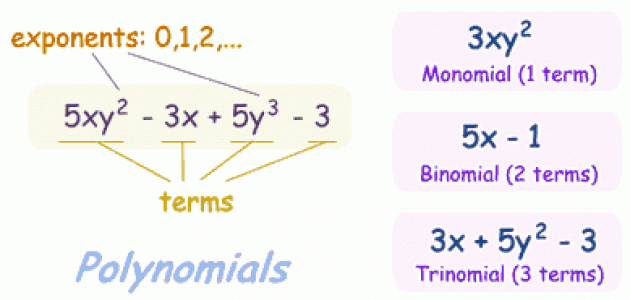# Difference between Polynomial and Monomial

Polynomial and monomial are two mathematical terms mostly used in algebra. In the context of algebra, they are the same thing and are interchangeable to some extent; however, there are a few differences as well, understanding which will help improve your ability to learn the algebraic functions.

The basic difference between these two algebraic terms is that a polynomial, as the name (poly) suggests, is a broader term as compared to monomial. All monomials are polynomials, but not all polynomials are monomials. You can also say that a monomial is a subset of a polynomial. In simple words, a monomial is a polynomial which has only one term. Two or more monomials can be added to get a polynomial, whereas to make a monomial from two or more polynomials, you always need to subtract the terms.

The terms comprising a monomial or a monomial itself, must have a real number (0, +1, +2, +3…) and a variable (e.g. x, y, z). The real number can be an integer, a whole number or a fraction. While the terms in a monomial are always separated by a multiplication sign, the terms in a polynomial are always separated by addition or subtraction signs. A multiplication sign between the different terms of a polynomial will make it a monomial with a higher degree of variables. Both polynomials and monomials can have more than one variable.

### Instructions

• 1

Monomial

A monomial is a term comprising a real number and one or more variables with whole number exponents.  For instance, where x, y and z are the variables, a, b and c are the non negative powers of these variables. The constant term in this example is 1. Furthermore, a variable with exponent zero becomes equal to 1. To add or subtract two monomials, both the terms should have similar variables, with the same exponents. Monomials with different variables or similar variables with varying exponents cannot be added or subtracted; instead they combine to form a polynomial. However, monomials with different variables can by multiplied, and the resulting term will also be a monomial.

Image courtesy: shmoop.com• 2

Polynomial

A polynomial is a combination of two or more monomials, detached by an addition or subtraction sign. Each monomial in the expression is called a term of the polynomial. x2 + 5x + 6, and x5 - 3x + 8 are examples of polynomials. A polynomial can be a monomial, binomial or trinomial, depending upon the number of terms present in it.

Image courtesy: mathsisfun.com•